Functions and CALL Routines

# CONVXP Function

Returns the convexity for a periodic cash flow stream, such as a bond.
 Category: Financial

## Syntax

 CONVXP(A,c,n,K,k0,y)

### Arguments

A

specifies the par value.

 Range: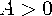c

specifies the nominal per-period coupon rate, expressed as a fraction.

 Range: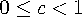n

specifies the number of coupons per period.

 Range: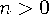and is an integer
K

specifies the number of remaining coupons.

 Range: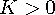and is an integer
k0

specifies the time from the present date to the first coupon date, expressed in terms of the number of periods.

 Range: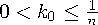y

specifies the nominal per-period yield-to-maturity, expressed as a fraction.

 Range: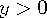The CONVXP function returns the value
where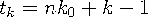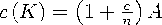and where
In the following example, the CONVXP function returns the convexity of a bond that has a face value of 1000, an annual coupon rate of 0.01, 4 coupons per year, and 14 remaining coupons. The time from settlement date to next coupon date is 0.165, and the annual yield-to-maturity is 0.08.

```data _null_;
y=convxp(1000,.01,4,14,.33/2,.08);
put y;
run;```

The value that is returned is 11.729001987.Previous Page | Next Page | Top of Page